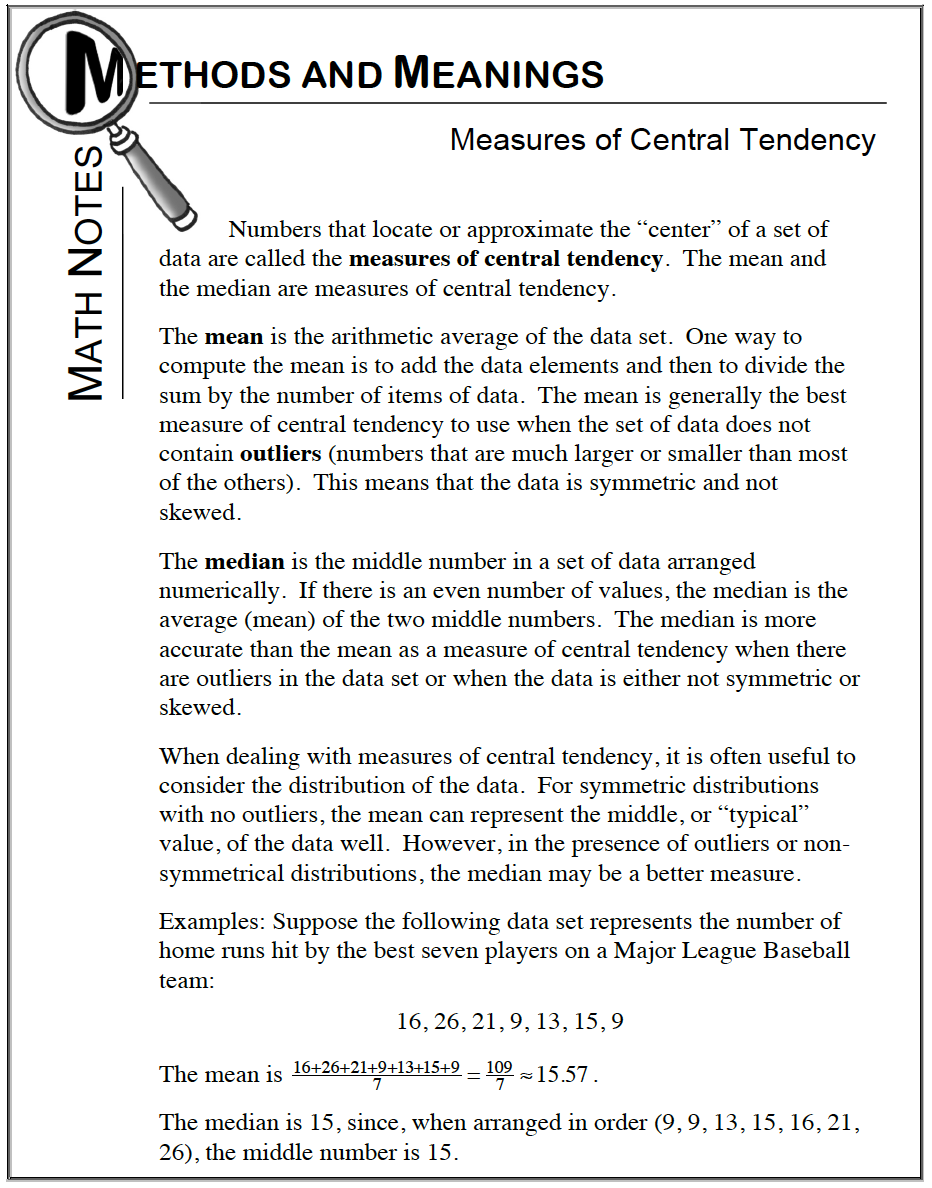Home > CC2MN > Chapter cc23 > Lesson cc23.2.4 > Problem3-76

3-76.

Find the mean, range, and median of the values: $12$, $4$, $-2$, $0$, $9$, $-2$ , $1$, $7$, $8$, $2$.  Recall that the range is calculated by finding the difference between the largest and smallest data values.  3-76 HW eTool (CPM).  Homework Help ✎

The range of a set of data is the difference between the highest and the lowest values.

The range of a set of data is the difference between the highest and the lowest values.